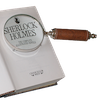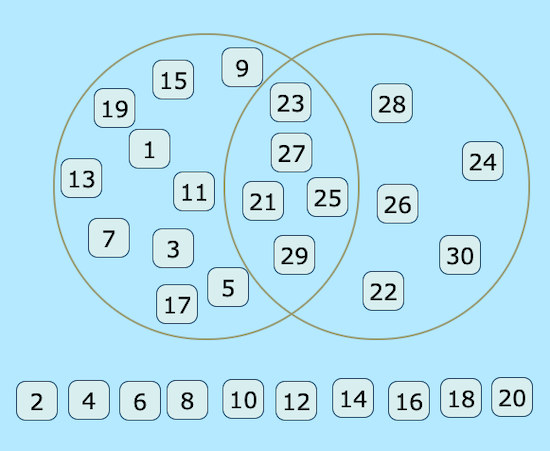#### You may also like### Number Round Up

Arrange the numbers 1 to 6 in each set of circles below. The sum of each side of the triangle should equal the number in its centre.### Number Detective

Follow the clues to find the mystery number.### Grouping Goodies

Pat counts her sweets in different groups and both times she has some left over. How many sweets could she have had?

# Venn Diagrams

##### Age 5 to 11Challenge Level

Take a look at the interactivity below. Before you try anything, have a think about these questions:

What do you see?
What do you wonder?

We call this way of sorting information a Venn diagram (named after the mathematician John Venn).

Can you drag the numbers from 1 to 30 into their correct places in the Venn diagram?
How do you know where to put each number?

Here is another one for you to try.
How do you know where to put each number this time?

If you would prefer to work away from a screen, you could print off these sheets, which have a copy of each Venn diagram on them.

Here is a screenshot (a picture) of the interactivity, but the labels of the Venn diagram are missing.

What could the labels be?Here is another version of the interactivity for you to try.

What do you notice this time?